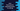# Java program to find count of words and find repeating words in a String### Java program to print count of each word in a string and find repeating words :

In this tutorial, we will learn how to print the count of each word in a string using Java. The user will first enter the string, and then we will find the count and print out the result. Let’s take a look at the program first :

### Java Program :

``````import java.util.HashMap;
import java.util.Map;
import java.util.Scanner;

public class Main {

public static void main(String[] args) {
//1
String inputString;

//2
Scanner scanner = new Scanner(System.in);

//3
int count;

//4
System.out.println("Enter a string : ");

//5
inputString = scanner.nextLine();

//6
String[] wordsArray = inputString.split("\\s+");

//7
HashMap<String, Integer> map = new HashMap<>();

//8
for (String word : wordsArray) {
//9
if (map.containsKey(word)) {
count = map.get(word);
map.put(word, count + 1);
} else {
map.put(word, 1);
}
}

//10
for (Map.Entry<String, Integer> entry : map.entrySet()) {
System.out.println(entry.getKey() + " : " + entry.getValue());
}

}

}``````

### Explanation :

1. Create one String object to store the user input string: inputString.
2. Create one Scanner object to read the user input.
3. Create one integer variable to store the current count of a word.
4. Ask the user to enter a string.
5. Read the string and store it in variable inputString.
6. Split the string into an array of words using split() function. We are passing \s+ as regex to this function. That means words will be separated by blank space.
7. Create one HashMap to store each word and count of that word. Each word is stored as key and the count as value.
8. Use one for loop to scan each word in the array. We are reading words one by one.
9. Check if the HashMap contains that word or not. If the word is already stored in the map, increase the count and store the updated count as value for that word. Else store value as 1 for that key (word).
10. After all words are scanned, run one for loop to read all key-value pairs. Print all words and count for each word.

### Sample Output :

``````Enter a string :
This is a string This
a : 1
string : 1
This : 2
is : 1

Enter a string :
one two three one two three
one : 2
two : 2
three : 2``````